Type
Solution Manual
Book Title
N/A
ISBN 13
N/A

### 978-0078025754 Appendix B Solution Manual

March 26, 2020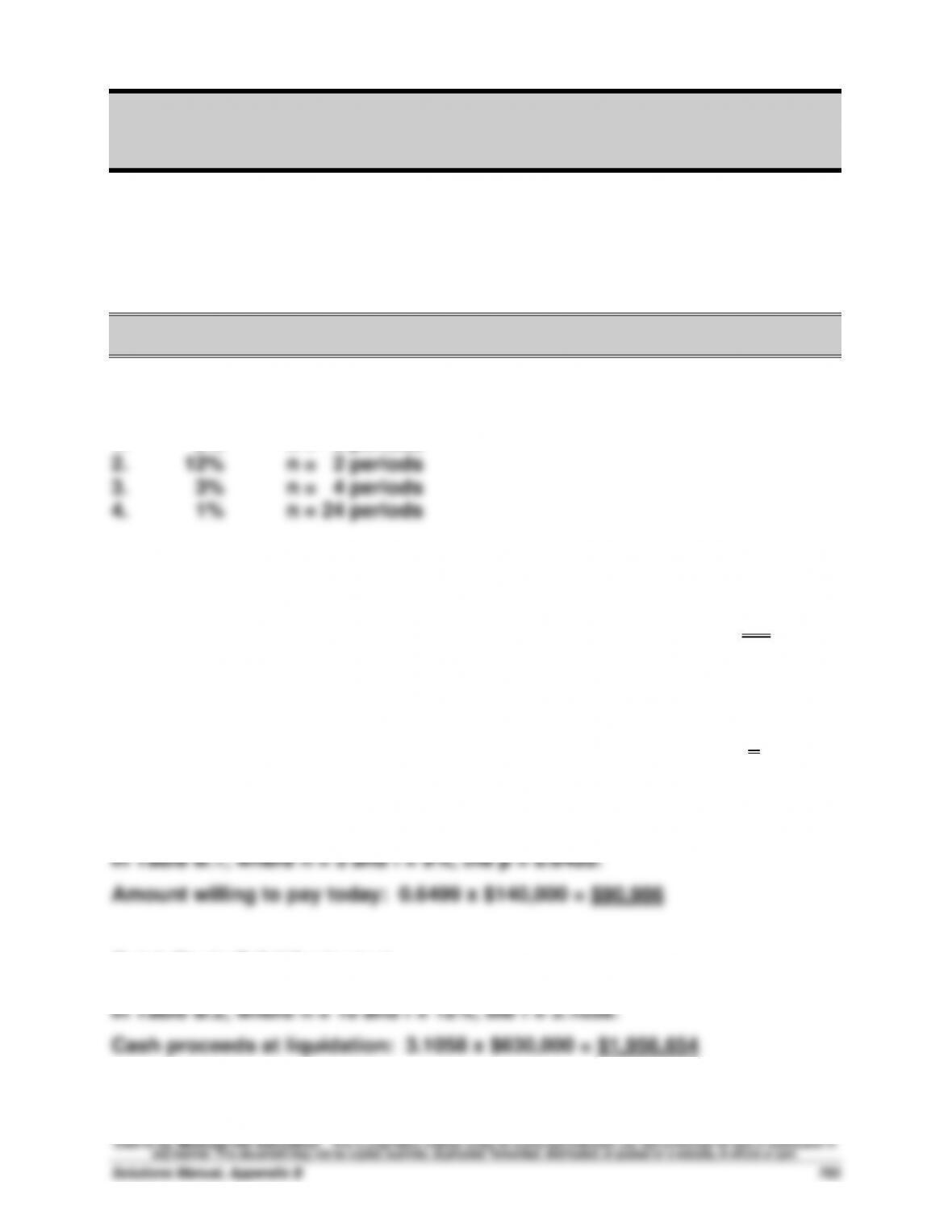Appendix B
Time Value of Money
QUICK STUDIES
Quick Study B-1 (10 minutes)
1.
2%
n = 8 periods
Quick Study B-2 (10 minutes)
In Table B.1, where n = 15 and p = \$2,745/\$10,000 = 0.2745, the i = 9%.
Quick Study B-3 (10 minutes)
In Table B.1, where i = 6% and p = \$6,651/\$10,000 = 0.6651, the n = 7.
Quick Study B-4 (10 minutes)
Quick Study B-5 (10 minutes)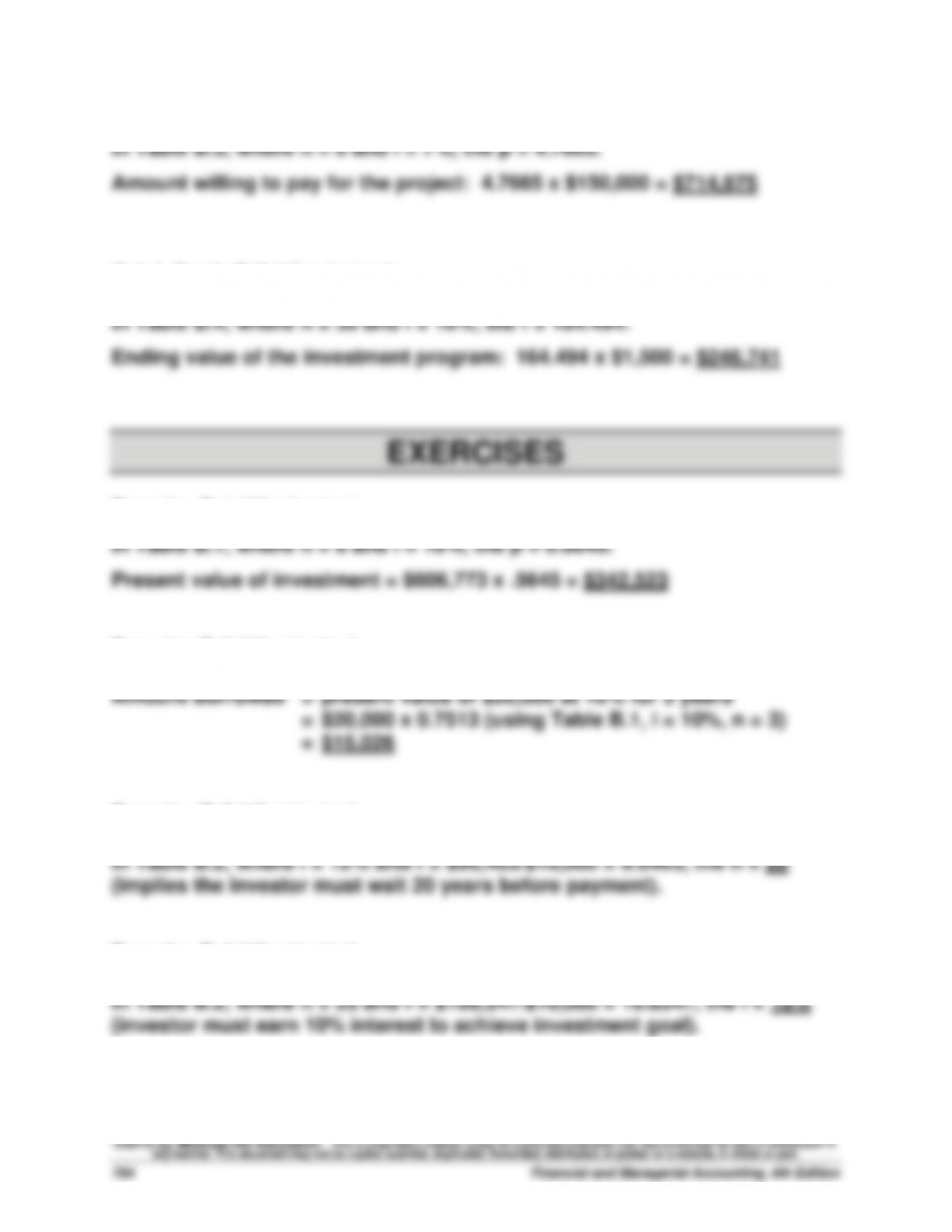Quick Study B-6 (10 minutes)
Quick Study B-7 (10 minutes)
Exercise B-1 (15 minutes)
Exercise B-2 (15 minutes)
Exercise B-3 (10 minutes)
Exercise B-4 (10 minutes)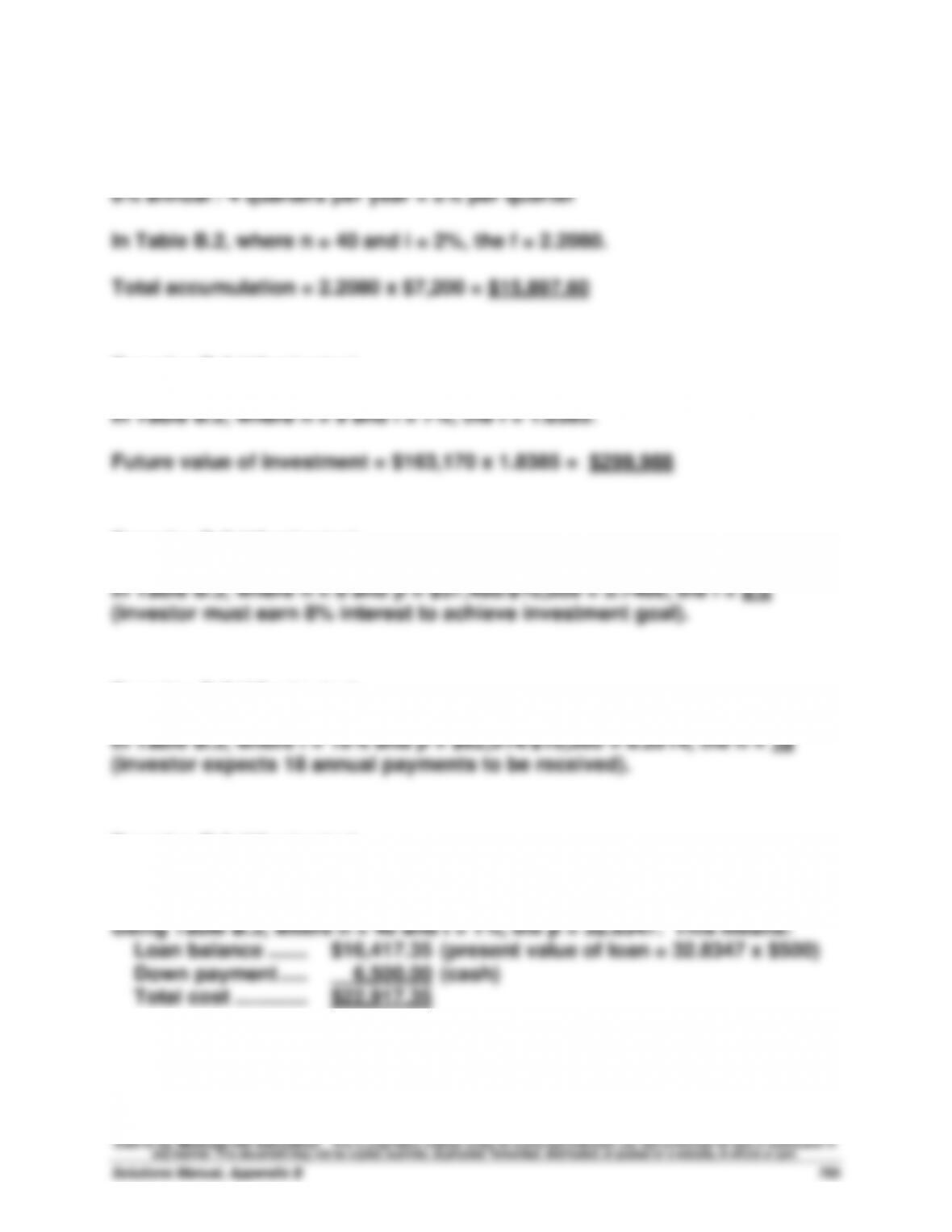Exercise B-5 (15 minutes)
10 years x 4 quarters = 40 interest periods
Exercise B-6 (15 minutes)
Exercise B-7 (10 minutes)
Exercise B-8 (10 minutes)
Exercise B-9 (10 minutes)
Interest rate per period = 12% annual / 12 months per year = 1% per month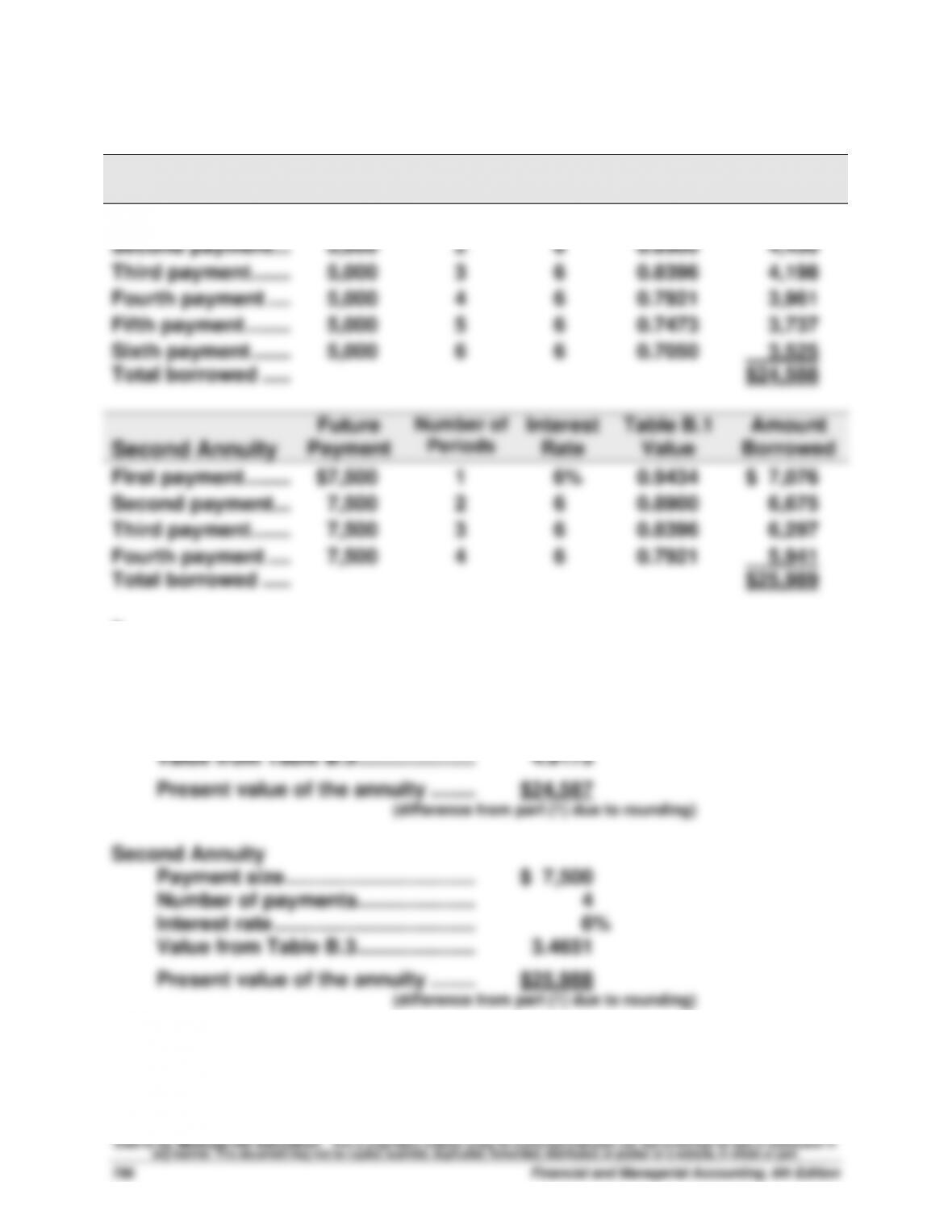Exercise B-10 (25 minutes)
1.
First Annuity
Future
Payment
Number of
Periods
Interest
Rate
Table B.1
Value
Amount
Borrowed
First payment ........
\$5,000
1
6%
0.9434
\$ 4,717
2.
First Annuity
Payment size .......................................
\$ 5,000
Number of payments ..........................
6
Interest rate .........................................
6%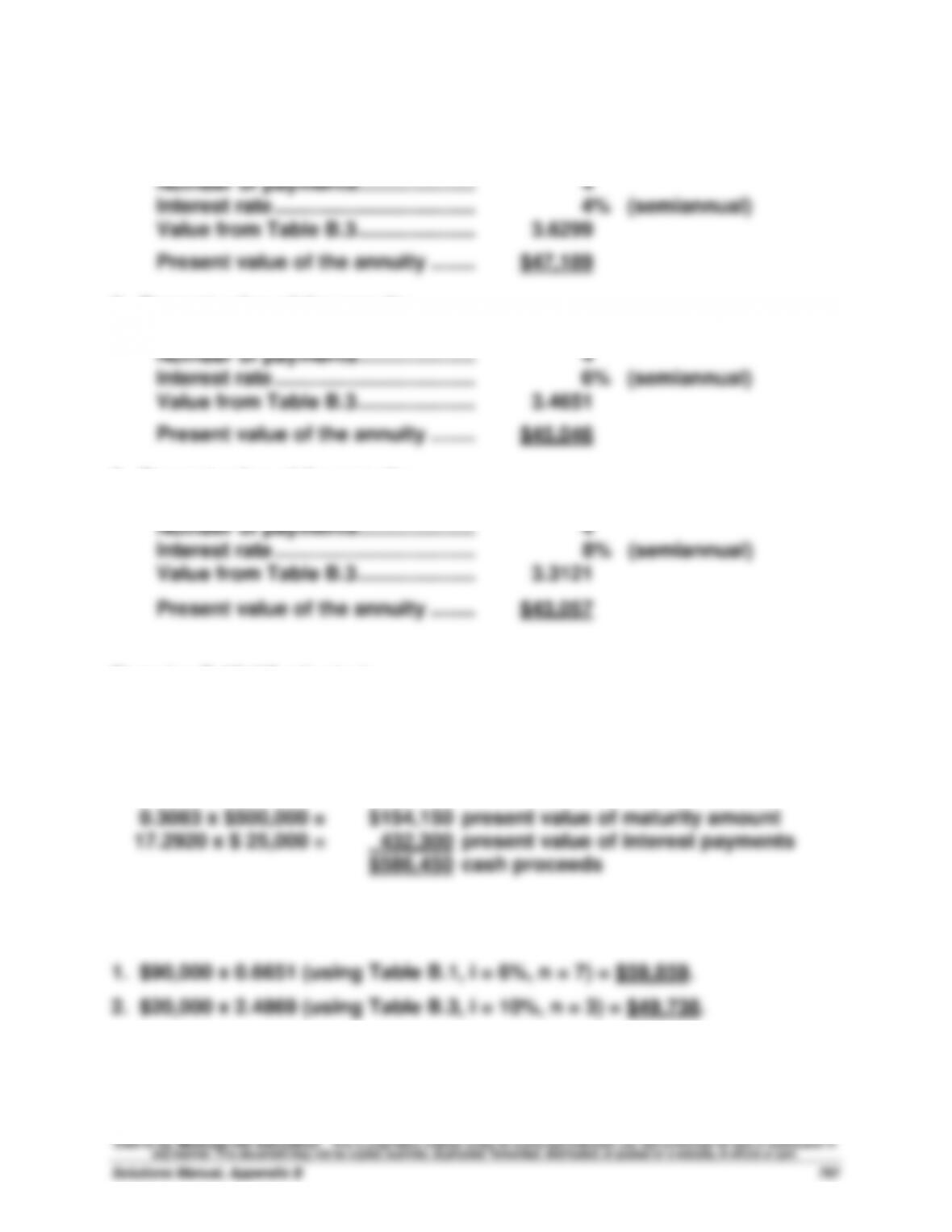Exercise B-11 (30 minutes)
1. Present value of the annuity
Payment size .......................................
\$13,000
2. Present value of the annuity
Payment size .......................................
\$13,000
3. Present value of the annuity
Payment size .......................................
\$13,000
Exercise B-12 (15 minutes)
Semiannual interest payment = \$500,000 x 10% x 1/2 = \$25,000
Using Table B.1, where n = 30 and i = 4%, the p = 0.3083 (Principal payment)
Using Table B.3, where n = 30 and i = 4%, the p = 17.2920 (Interest payments)
Exercise B-13 (15 minutes)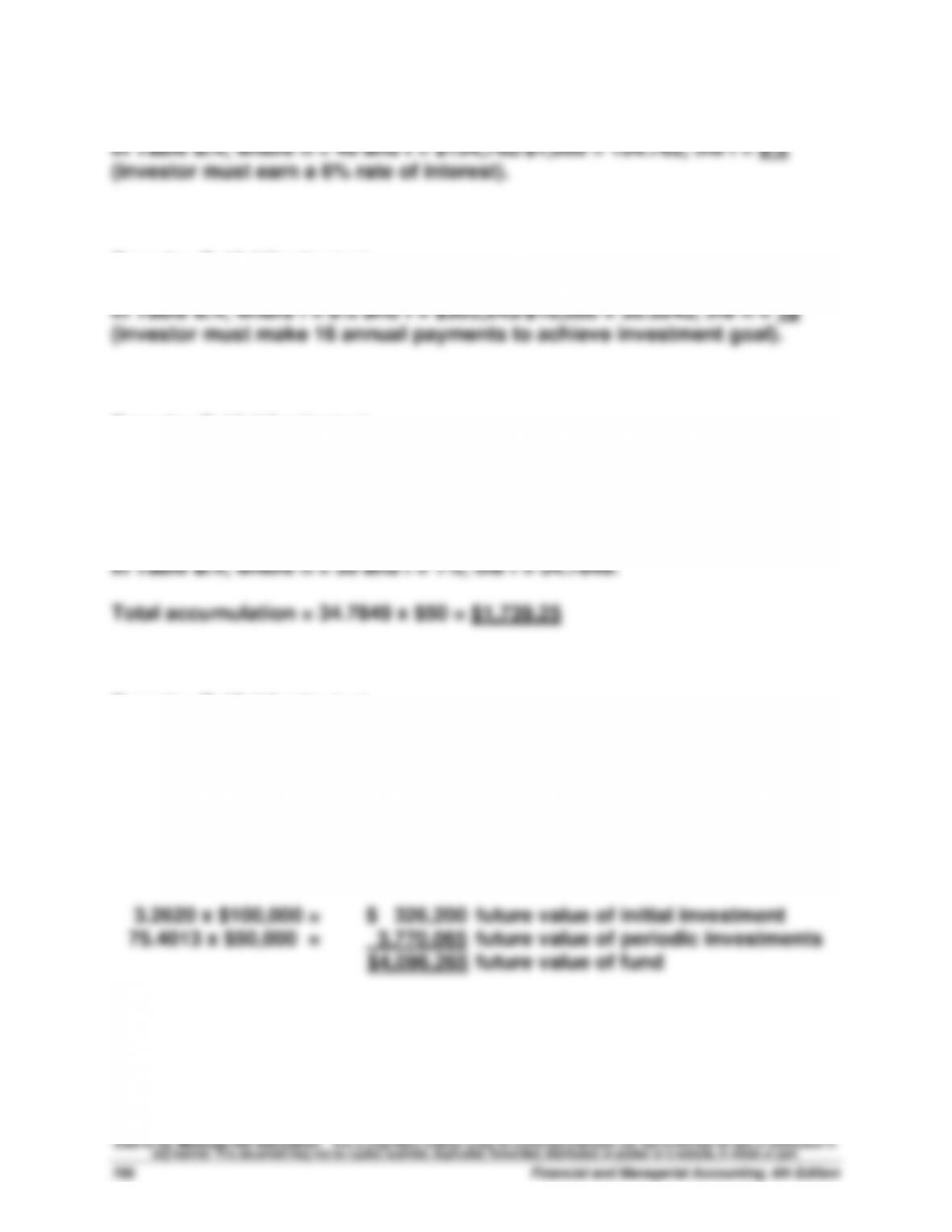Exercise B-14 (10 minutes)
Exercise B-15 (10 minutes)
Exercise B-16 (15 minutes)
12% annual / 12 months per year = 1% per month
2.5 years x 12 months per year = 30 total months
Exercise B-17 (15 minutes)
10 years x 4 quarters per year = 40 total quarters
12% annual / 4 quarters per year = 3% per quarter
In Table B.2, where n = 40 and i = 3%, the f = 3.2620.
In Table B.4, where n = 40 and i = 3%, the f = 75.4013.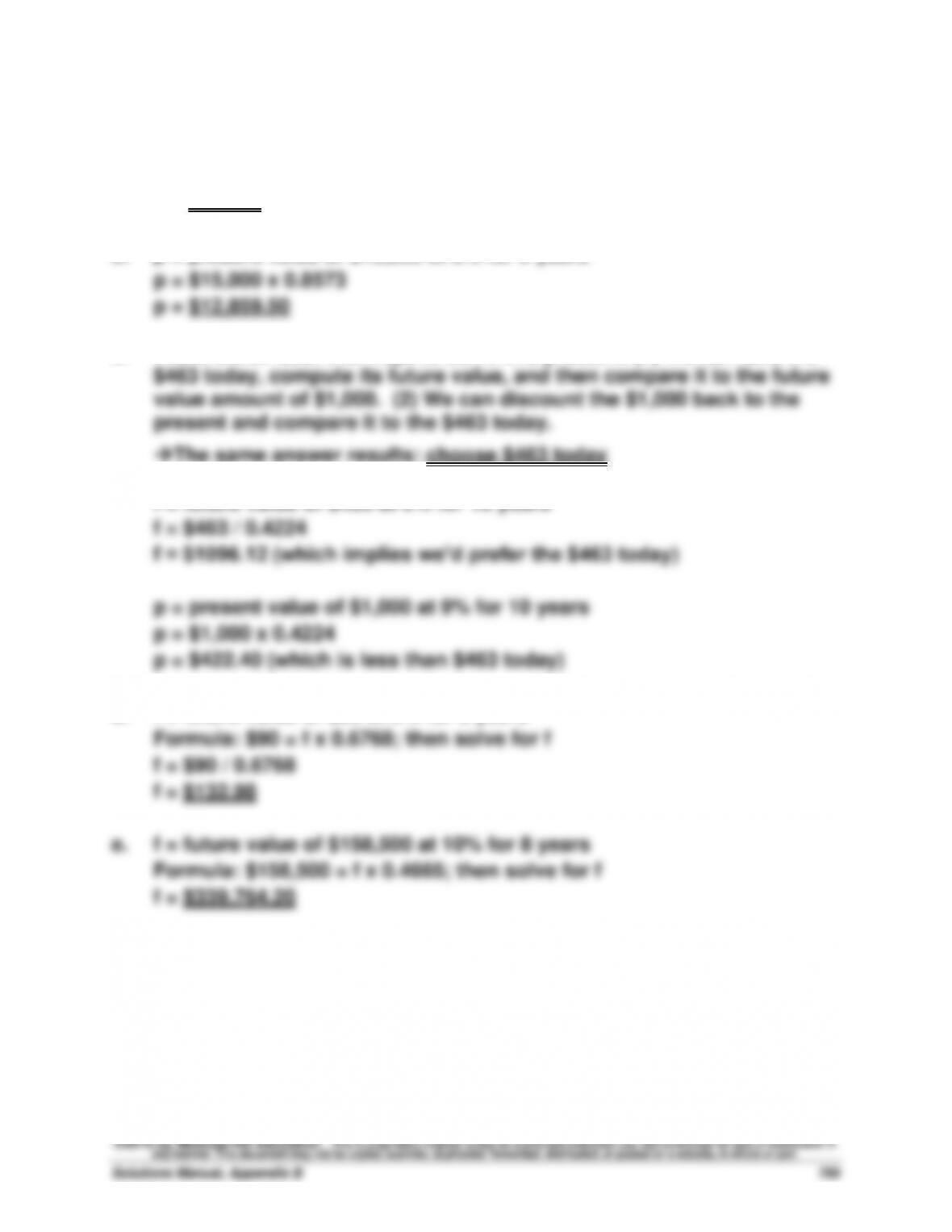Exercise B-18 (10 minutes)
a. p = present value of \$60,000 at 9% for 4 years
p = \$60,000 x 0.7084
p = \$42,504
b. p = present value of \$15,000 at 8% for 2 years
c. There are at least two ways to solve this problem. (1) We can take the
The same answer results: choose \$463 today
f = future value of \$463 at 9% for 10 years
d. f = future value of \$90 at 5% for 8 years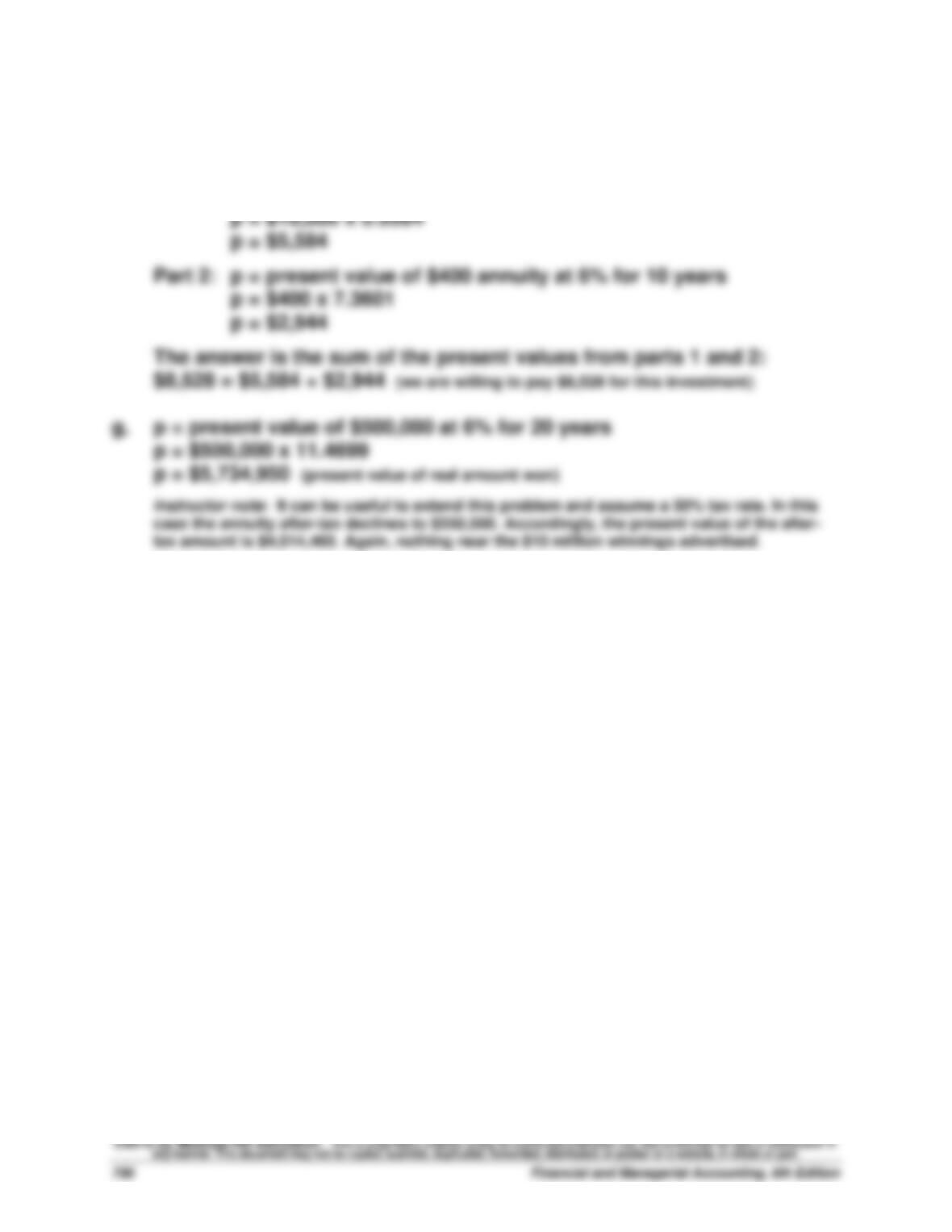Exercise B-18 (concluded)
f. There are two aspects to this problem: a present value of a lump sum
part and a present value of an annuity part.
Part 1: p = present value of \$10,000 at 6% for 10 years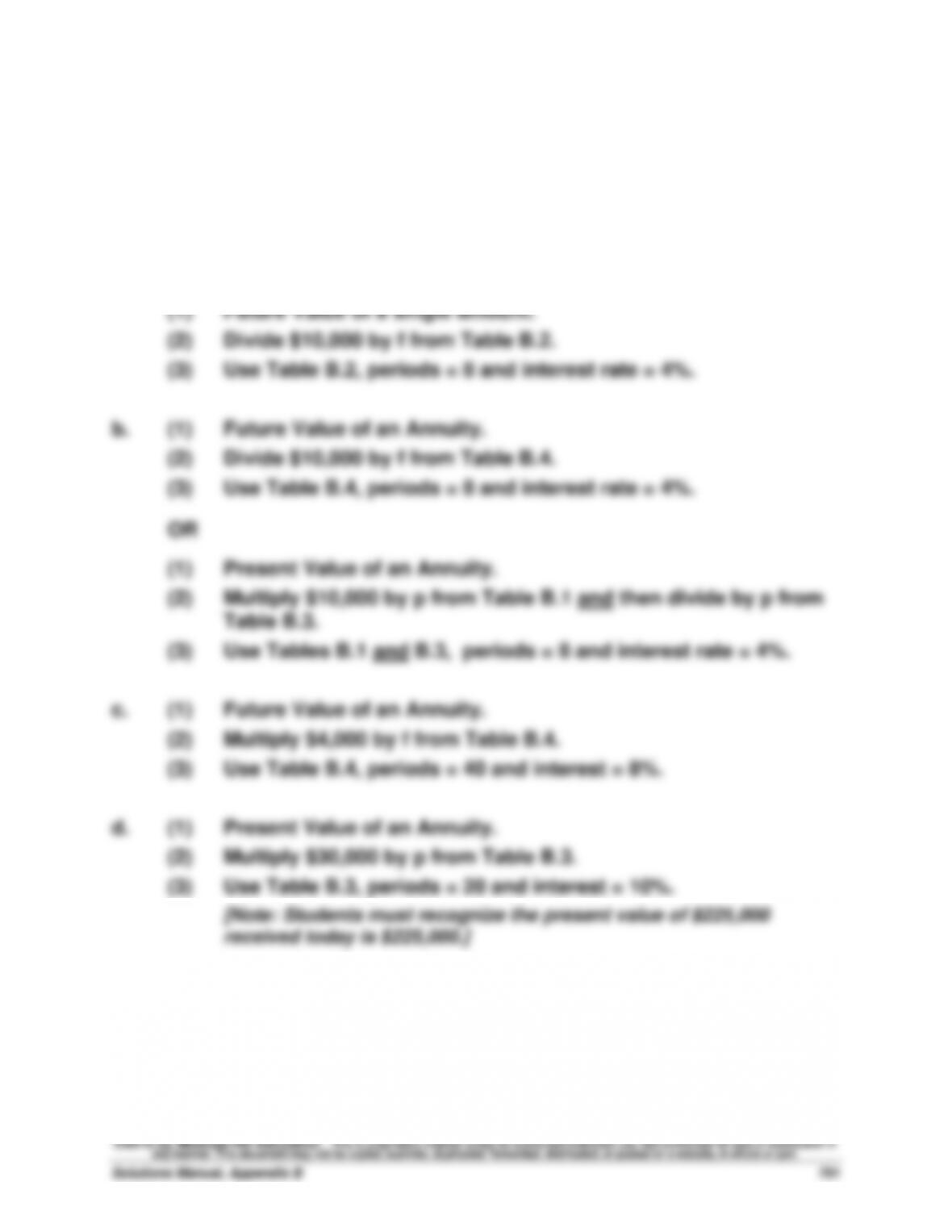Exercise B-19 (20 minutes)
a. (1) Present Value of a single amount.
(2) Multiply \$10,000 by p from Table B.1.
(3) Use Table B.1, periods = 8 and interest rate = 4%.
OR